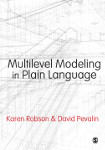# Multilevel Modeling in Plain Language

Have you been told you need to do multilevel modeling, but you can’t get past the forest of equations? Do you need the techniques explained with words and practical examples so they make sense? Help is here! This book unpacks these statistical techniques in easy-to-understand language with fully annotated examples using the statistical software Stata. The techniques are explained without reliance on equations and algebra so that new users will understand when to use these approaches and how they are really just special applications of ordinary regression. Using real life data, the authors show you how to model random intercept models and random coefficient models for cross-sectional data in a way that makes sense and can be retained and repeated. This book is the perfect answer for anyone who needs a clear, accessible introduction to multilevel modeling.

•••